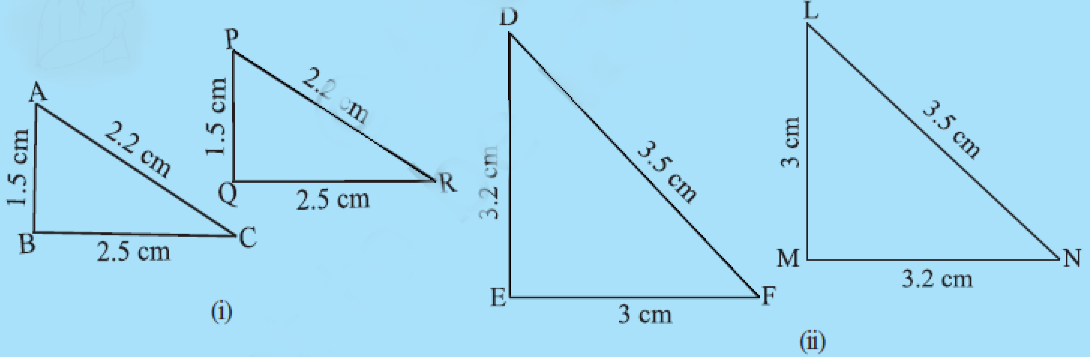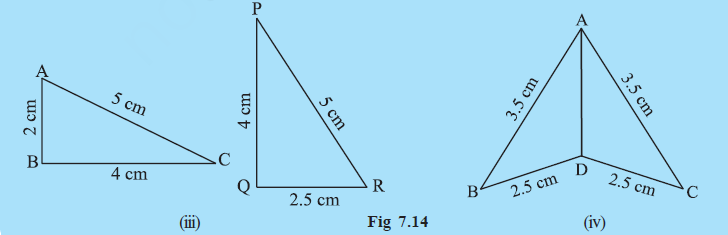Q

# In Fig 7.14, lengths of the sides of the triangles are indicated. By applying the SSS congruence rule, state which pairs of triangles are congruent. In case of congruent triangles, write the result in symbolic form:

1. In Fig 7.14, lengths of the sides of the triangles are indicated. By applying the SSS congruence rule, state which pairs of triangles are congruent. In case of congruent triangles, write the result in symbolic form:Views

i) Since

AB = PQ

BC = QR

CA = PR

So, by SSS congruency rule both triangles are congruent to each other.

ii) Since,

ED = MN

DF = NL

FE = LM

So, by SSS congruency rule both triangles are congruent to each other.

.

iii) Since

AC = PR

BC = QR But

So the given triangles are not congruent.

iv) Since,

AB = AC

BD = CD

So, By SSS Congruency rule, they both are congruent to each other.

.

Exams
Articles
Questions